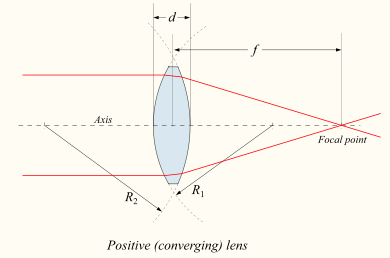## Lensmaker Equation Calculator

 Radius of Curvature of the First Surface: Radius of Curvature of the Second Surface: Refractive Index of Lens Material: gmol/liter Refractive Index of Ambient Medium: kelvin Focal Length:

A lens is an optical device which transmits and refracts light, The Lens maker equation calculate the Focal length.### Lensmaker's equation formula

1/f = (n1/nm-1) * (1/r1-1/r2)

Where n1 -Refractive Index of Lens Material, nm -Refractive Index of Ambient Medium, r1 -Radius of Curvature of the First Surface, r2 - Radius of Curvature of the Second Surface

For example, when Radius of Curvature of the First Surface is 5, Radius of Curvature of the Second Surface is 4, Refractive Index of Lens Material is 1 gmol/liter, Refractive Index of Ambient Medium is 2 kelvin, then the Focal Length is 0.024999999999999994.

Thinkcalculator.com provides you helpful and handy calculator resources.